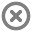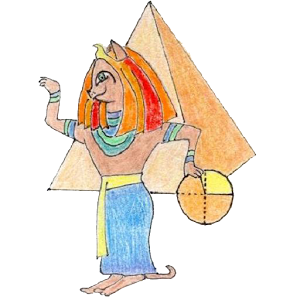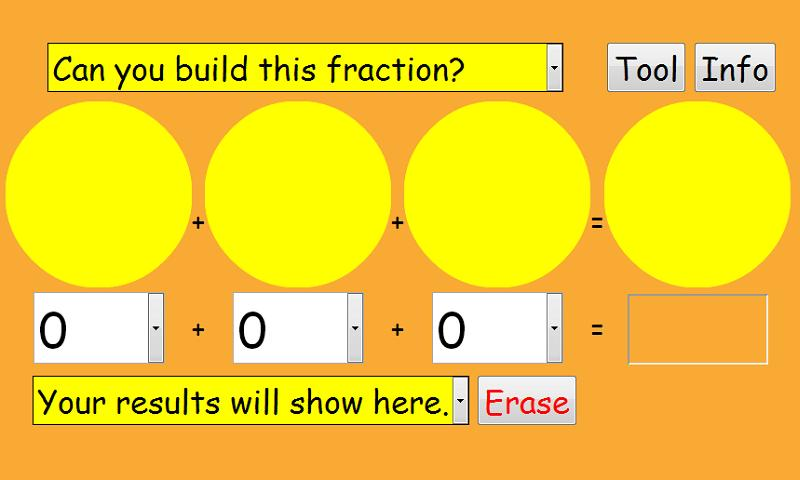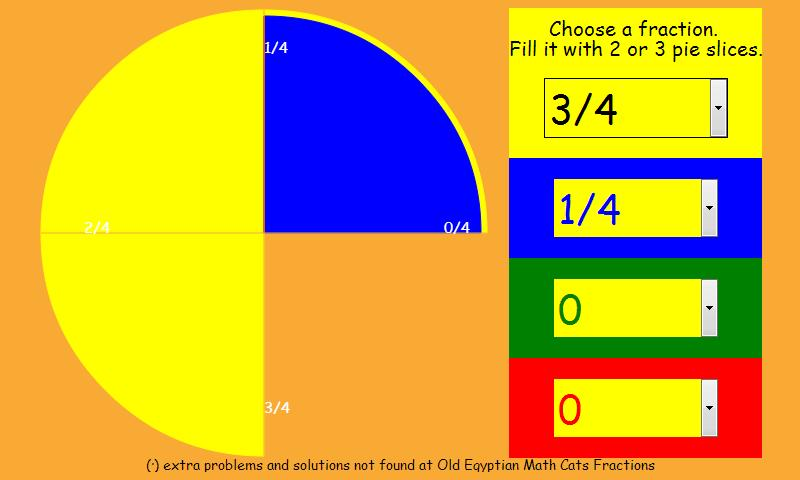Loading...## Info## Error# Old Egyptian Fractions

by MATHCATS

Application ( 10MB )

Free## Description

This is the android version of the Mathcats Old Egyptian Fractions:

Old Egyptian Math Cats knew fractions like 1/2 or 1/4 (one piece of a pie).
But to make fractions like 3/4, they had to add pieces of pies like 1/2 + 1/4 = 3/4.
Old Egyptian Math cats never repeated the same fraction when adding.
They never wrote: 1/4 + 1/4 + 1/4 = 3/4

In ancient Egypt, they wrote fractions with numerator 1 or as a sum of fractions with numerator 1. So the fraction 2/3 would be 1/2 +1 / 6. It has a certain logic if we consider a particular case: if we divide two loaves among three people will give each bread half (1/2) and a third of the bread is left half (1/6).

How it works:

Choose puzzles from the list on the top. ( * = easier, **** = very hard.)
Add 2 or 3 fraction pieces below.
After you find one solution, the puzzle is marked "Solved."
Can you find more solutions? (Click to see them listed on the bottom.)

You can play with the internet version of Old Egyptian Fractions at:
http://www.mathcats.com/explore/oldegyptianfractions.html